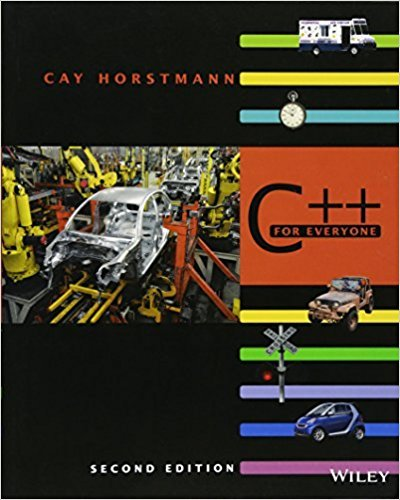×
Get Full Access to C++ For Everyone - 2 Edition - Chapter 6 - Problem R6.10
Get Full Access to C++ For Everyone - 2 Edition - Chapter 6 - Problem R6.10

×

# Trace the flow of the element separator loop in Section 6.2.5 with the givenISBN: 9780470927137 356

## Solution for problem R6.10 Chapter 6

C++ for Everyone | 2nd Edition

• Textbook Solutions
• 2901 Step-by-step solutions solved by professors and subject experts
• Get 24/7 help from StudySoup virtual teaching assistantsC++ for Everyone | 2nd Edition

4 5 1 316 Reviews
18
3
Problem R6.10

Trace the flow of the element separator loop in Section 6.2.5 with the given example.Show two col umns, one with the value of i and one with the output.

Step-by-Step Solution:
Step 1 of 3

ENGR 121 B Lab Notes for 10/24/2016 Spencer Kociba ● scalarctneeds to have scalar.^vector ● vector(logical vector) ○ Will find every value for whom’s element equals 1 in the logical vector ● Looping repeats the action for every element in a vector ● Example of nested for loops ○ For m=1:3 ■ For n=-1:-1:-3 ● fprintf(‘m=%d, n=%d\n, m,n) ■ End ○ End ○ >> %Will give all combinations created by the for loop (9 in this one) ■ m=1, n=-1 ■ m=1, n=-2 ■ … ■ m=3, n=-3

Step 2 of 3

Step 3 of 3

## Discover and learn what students are asking

Calculus: Early Transcendental Functions : Iterated Integrals and Area in the Plane
?In Exercises 1 - 10, evaluate the integral. $$\int_{x}^{x^{2}} \frac{y}{x} d y$$

Statistics: Informed Decisions Using Data : Comparing Three or More Means (One-Way Analysis of Variance)
?Which Delivery Method Is Best? At a community college, the mathematics department has been experimenting with four different delivery mechanisms for c

Statistics: Informed Decisions Using Data : Testing the Significance of the Least-Squares Regression Model
?Why don’t we conduct inference on the linear correlation coefficient?

Unlock Textbook Solution